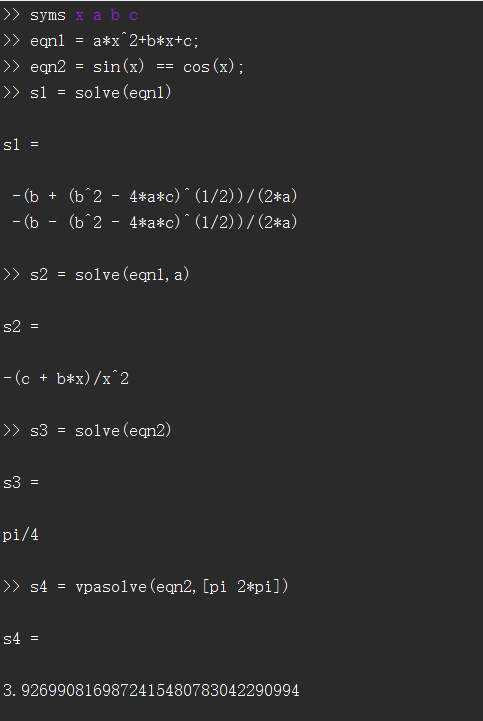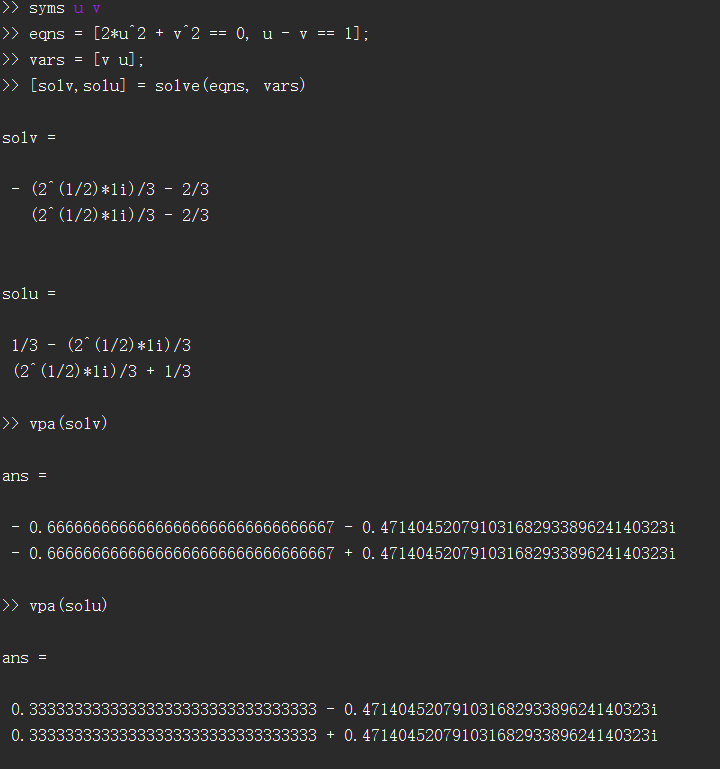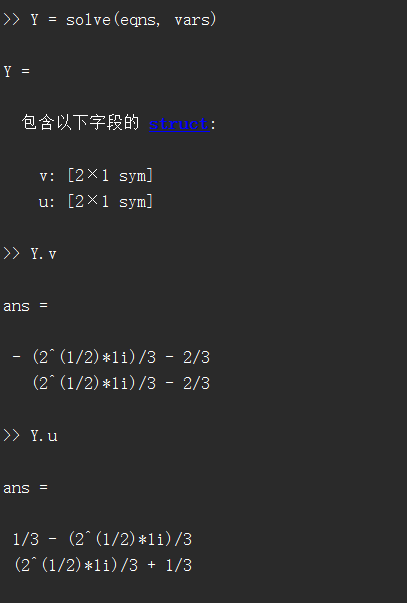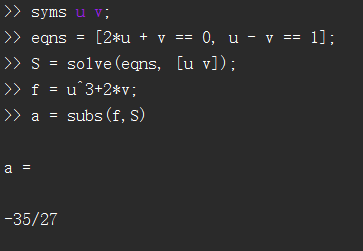• 定义方程组 eq1=y==3*x+5; eq2=y==4*x+6; 求解方程组 s=solve(eq1,eq2,[x,y]); s.x s.y 注意老版本使用的是 s=solve('eq1','eq2','x','y') 这种表示已经淘汰了. 另外s.x是sym格式, 如果拿到真正的值 double(s....

定义位置变量

syms x y


定义方程组

eq1=y==3*x+5;
eq2=y==4*x+6;


求解方程组

s=solve(eq1,eq2,[x,y]);
s.x
s.y

注意老版本使用的是
s=solve('eq1','eq2','x','y')

这种表示已经淘汰了.
另外s.x是sym格式, 如果拿到真正的值
double(s.x)

即可.


展开全文• MATLAB作为科研工作者的忠实伙伴，解各种复杂方程的性能与其他工具相比，自然不逞多让。本期盘点MATLABsolve和fsolve两个函数在解方程中的优劣。
MATLAB作为科研工作者的忠实伙伴，解各种复杂方程的性能与其他工具相比，自然不逞多让。本期盘点MATLAB中solve和fsolve两个函数在解方程中的优劣。
1. solve函数
语法：
S = solve(eqn,var)
S = solve(eqn,var,Name,Value)
Y = solve(eqns,vars)
Y = solve(eqns,vars,Name,Value)
[y1,...,yN] = solve(eqns,vars)
[y1,...,yN] = solve(eqns,vars,Name,Value)
[y1,...,yN,parameters,conditions] = solve(eqns,vars,'ReturnConditions',true)

描述：
S = solve(eqn,var) 求解变量var的方程eqn 。如果未指定var, symvar 函数将确定要为其求解的变量。例如, solve(x + 1 == 2, x)解决等式 x + 1 = 2为x .
例子
S = solve(eqn,var,Name,Value) 使用由一个或多个Name,Value对参数指定的附加选项。
例子
Y = solve(eqns,vars) 解决了方程组eqns的变量vars , 并返回一个结构, 包含解决方案。如果不指定vars, 则solve使用 symvar 查找要解决的变量。在这种情况下, symvar发现的变量数等于等式的个数eqns.
例子
Y = solve(eqns,vars,Name,Value) 使用由一个或多个Name,Value对参数指定的附加选项.
例子
[y1,...,yN] = solve(eqns,vars) 解决了变量vars的eqns方程组。解决方案被分配给y1,...,yN的变量。如果不指定变量, solve使用symvar查找要解决的变量。在这种情况下, symvar发现的变量数等于输出参数的个数N.
[y1,...,yN] = solve(eqns,vars,Name,Value) 使用由一个或多个名称指定的附加选项Name,Value对参数。
例子
[y1,...,yN,parameters,conditions] = solve(eqns,vars,'ReturnConditions',true) 返回指定解决方案中的参数和解决方案条件的附加参数parameters和conditions。

solve 函数的局限性

对于非多项式方程，只能求出一个解
对于稍许复杂的方程，求解结果出现很大误差
求解复杂的多项式方程时，可能会产生错误的求解结果
求解复杂的多项式方程时，可能无法求解，且非常耗时
求解超越方程时，只能返回一个解；
求解超越方程时，可能返回错误解；

2. fsolve函数
语法(仅列出两种)
x = fsolve(fun,x0)
[x,fval,exitflag] = fsolve(fun,x0,options)
fun: 函数，用于定义方程(组)
x0: 计算初值
x: 求解结果(方程的根)
fval: 将求解结果x 带入方程(组) fun，对应的值，即fun(x)
exitflag: 返回方程组求解结果的状态(详见help 文档)
options: 方程的求解设置


MATLAB fsolve函数总结

fsolve可以求解方程(组) 的实数根和复数根
fsolve采用迭代的数值算法，速度快
给定不同的初值，可以求得不同的根(局部寻根)
初值给的不好，可能导致求解失败
关于初值如何给定的问题
a) 一元/ 二元方程(组)，通过图解法，可以得到根的个数，并粗略地估计出根的值，用做fsolve的初值
b) 根据方程组中变量的实际意义，合适地给出初值。例如，时间/ 长度/ 质量等物理量，应该大于0
c) 通过更多的练习和经验积累，自然会见多识广

总结：
尽量避免使用solve函数
尽可能使用fsolve求解数值解
如果觉得本文写的还不错的伙伴，可以给个关注一起交流进步，如果有在找工作且对阿里感兴趣的伙伴，也可以发简历给我进行内推：展开全文fsolve
• ## MATLAB解方程组

千次阅读 2019-01-21 23:13:17
解单一方程 S=solve(eqn,var) 解等式eqn关于自变量var的解。var省略则自动检测（默认为x）。其中eqn可以是等式，也可以是函数，若为函数，则默认为eqn=...解方程组 Y=solve(eqns,vars) eqns是方程组的符号向量，即[e...
解单一方程
S=solve(eqn,var)
解等式eqn关于自变量var的解。var省略则自动检测（默认为x）。其中eqn可以是等式，也可以是函数，若为函数，则默认为eqn==0的解。
S=vpasolve(eqn,[a b])
计算eqn在区间$[a,b]$上的解，但是只能是数不能含有符号参数。解方程组
Y=solve(eqns,vars)
eqns是方程组的符号向量，即$[eqn1,eqn2,...]$。var是未知数向量，Y是所求结果。如果只写一个Y，则返回的Y是结构体，如果将Y写成$[sol1,sol2,...]$的形式，则每个元素为var中的解。方程组的解带入函数
当方程组的解是结构体Y时，要计算函数$f$的值，可以使用语句：
$A=subs(f,Y)$，即可将方程得到的解带入$f$。展开全文• ## matlab解方程、方程组

万次阅读 多人点赞 2016-06-23 17:11:03
最近有多人问如何用matlab解方程组的问题，其实在matlab中解方程组还是很方便的，例如，对于代数方程组Ax=b(A为系数矩阵，非奇异)的求解，MATLAB中有两种方法： (1)x=inv(A)*b — 采用求逆运算解方程组；  (2)x=A...
1、解方程、方程组
x^2-4=12,求x：
syms x;
f=x^2-4-12;
solve(f)

最近有多人问如何用matlab解方程组的问题，其实在matlab中解方程组还是很方便的，例如，对于代数方程组Ax=b(A为系数矩阵，非奇异)的求解，MATLAB中有两种方法：
(1)x=inv(A)*b — 采用求逆运算解方程组；  (2)x=A\B — 采用左除运算解方程组
PS：使用左除的运算效率要比求逆矩阵的效率高很多~
例:
x1+2x2=8
2x1+3x2=13
>>A=[1,2;2,3];b=[8;13];
>>x=inv(A)*b
x =
2.00
3.00

>>x=A\B
x =
2.00
3.00；
即二元一次方程组的解x1和x2分别是2和3。
对于同学问到的用matlab解多次的方程组，有符号解法，方法是：先解出符号解,然后用vpa(F,n)求出n位有效数字的数值解.具体步骤如下：
第一步:定义变量syms x y z ...;
第二步:求解[x,y,z,...]=solve('eqn1','eqn2',...,'eqnN','var1','var2',...'varN');
第三步:求出n位有效数字的数值解x=vpa(x,n);y=vpa(y,n);z=vpa(z,n);...。
如：解二（多）元二（高）次方程组：
x^2+3*y+1=0
y^2+4*x+1=0
解法如下：
>>syms x y;
>>[x,y]=solve('x^2+3*y+1=0','y^2+4*x+1=0');
>>x=vpa(x,4);
>>y=vpa(y,4);
结果是：
x =
1.635+3.029*i
1.635-3.029*i
-.283
-2.987
y =
1.834-3.301*i
1.834+3.301*i
-.3600
-3.307。
二元二次方程组，共4个实数根；
还有的同学问，如何用matlab解高次方程组（非符号方程组）？举个例子好吗？
解答如下：
基本方法是：solve(s1,s2,…,sn,v1,v2,…,vn)，即求表达式s1,s2,…,sn组成的方程组，求解变量分别v1,v2,…,vn。
具体例子如下：
x^2 + x*y + y = 3
x^2 - 4*x + 3 = 0
解法：
>> [x,y] = solve('x^2 + x*y + y =3','x^2 - 4*x + 3 = 0')
运行结果为
x =
1 3
y =
1 -3/2
即x等于1和3；y等于1和-1.5
或
>>[x,y] = solve('x^2 + x*y + y =3','x^2 - 4*x + 3= 0','x','y')
x =
1 3
y =
1 -3/2
结果一样，二元二方程都是4个实根。
通过这三个例子可以看出，用matlab解各类方程组都是可以的，方法也有多种，只是用到解方程组的函数，注意正确书写参数就可以了，非常方便。
citefrom:http://bbs.seu.edu.cn/pc/pccon.php?id=950&nid=14498&tid=0
2、变参数非线性方程组的求解
对于求解非线性方程组一般用fsolve命令就可以了，但是对于方程组中某一系数是变化的，该怎么求呢？
%定义方程组如下，其中k为变量
function F = myfun(x,k)
H=0.32;
Pc0=0.23;W=0.18;
F=[Pc0+H*(1+1.5*(x(1)/W-1)-0.5*(x(1)/W-1)^3)-x(2);
x(1)-k*sqrt(x(2))];
%求解过程
H=0.32;
Pc0=0.23;W=0.18;
x0 = [2*W; Pc0+2*H]; % 取初值
options = optimset('Display','off');
k=0:0.01:1; % 变量取值范围[0 1]
for i=1:1:length(k)
kk=k(i);
x = fsolve(@(x) myfun(x,kk), x0, options);%求解非线性方程组
x1(i)=x(1);
x2(i)=x(2);
end
plot(k,x1,'-b',k,x2,'-r');
xlabel('k')
legend('x1','x2')
cite from:http://forum.simwe.com/archiver/tid-836299.html
3、非线性方程数值求解
matlab里solve如何使用,是否有别的函数可以代替它.
matlab里我解y=9/17*exp(-1/2*t)*17^(1/2)*sin(1/2*17^(1/2)*t)=0这样的方程为什么只得到0这一个解,如何可以的到1/2*17^(1/2)*t=n*(pi)这样一族解??
在matlab里面solve命令主要是用来求解代数方程（即多项式）的解,但是也不是说其它方程一个也不能解，不过求解非代数方程的能力相当有限，通常只能给出很特殊的实数解。(该问题给出的方程就是典型的超越方程，非代数方程)
从计算机的编程实现角度讲，如今的任何算法都无法准确的给出任意非代数方程的所有解，但是我们有很多成熟的算法来实现求解在某点附近的解。matlab也不例外，它也只能给出任意非代数方程在某点附近的解，函数有两个：fzero和fsolve,具体用法请用help或doc命令查询吧。如果还是不行，你还可以将问题转化为非线性最优化问题，求解非线性最优化问题的最优解，可以用的命令有：fminbnd,fminsearch, fmincon等等。
*非线性方程数值求解
*单变量非线性方程求解
在MATLAB中提供了一个fzero函数，可以用来求单变量非线性方程的根。该函数的调用格式为：
z=fzero('fname',x0,tol,trace)
其中fname是待求根的函数文件名，x0为搜索的起点。一个函数可能有多个根，但fzero函数只给出离x0最近的那个根。tol控制结果的相对精度，缺省时取tol=eps，trace�指定迭代信息是否在运算中显示，为1时显示，为0时不显示，缺省时取trace=0。
例 求f(x)=x-10x+2=0在x0=0.5附近的根。
步骤如下：
(1) 建立函数文件funx.m。
function fx=funx(x)
fx=x-10.^x+2;
(2) 调用fzero函数求根。
z=fzero('funx',0.5)
z =
0.3758
**非线性方程组的求解
对于非线性方程组F(X)=0，用fsolve函数求其数值解。fsolve函数的调用格式为：
X=fsolve('fun',X0,option)
其中X为返回的解，fun是用于定义需求解的非线性方程组的函数文件名，X0是求根过程的初值，option为最优化工具箱的选项设定。最优化工具箱提供了20多个选项，用户可以使用optimset命令将它们显示出来。如果想改变其中某个选项，则可以调用optimset()函数来完成。例如，Display选项决定函数调用时中间结果的显示方式，其中‘off’为不显示，‘iter’表示每步都显示，‘final’只显示最终结果。optimset(‘Display’,‘off’)将设定Display选项为‘off’。
例 求下列非线性方程组在(0.5,0.5) 附近的数值解。
(1) 建立函数文件myfun.m。
function q=myfun(p)
x=p(1);
y=p(2);
q(1)=x-0.6*sin(x)-0.3*cos(y);
q(2)=y-0.6*cos(x)+0.3*sin(y);
(2) 在给定的初值x0=0.5,y0=0.5下，调用fsolve函数求方程的根。
x=fsolve('myfun',[0.5,0.5]',optimset('Display','off'))
x =
0.6354
0.3734
将求得的解代回原方程，可以检验结果是否正确，命令如下：
q=myfun(x)
q =
1.0e-009 *
0.2375 0.2957
可见得到了较高精度的结果。
citefrom:http://blog.sina.com.cn/s/blog_56ef652d0100ebew.html
4、fsolve函数解方程
[X,FVAL,EXITFLAG,OUTPUT,JACOB]=FSOLVE(FUN,X0,...) returns the
Jacobian of FUN at X.
Examples
FUN can be specified using @:
x = fsolve(@myfun,[2 3 4],optimset('Display','iter'))
where myfun is a MATLAB function such as:
function F = myfun(x)
F = sin(x);
FUN can also be an anonymous function:
x = fsolve(@(x) sin(3*x),[1 4],optimset('Display','off'))
If FUN is parameterized, you can use anonymous functions to capturethe
problem-dependent parameters. Suppose you want to solve the systemof
nonlinear equations given in the function myfun, which isparameterized
by its second argument c. Here myfun is an M-file function suchas
function F = myfun(x,c)
F = [ 2*x(1) - x(2) - exp(c*x(1))
-x(1) + 2*x(2) - exp(c*x(2))];
To solve the system of equations for a specific value of c, firstassign the
value to c. Then create a one-argument anonymous function thatcaptures
that value of c and calls myfun with two arguments. Finally, passthis anonymous
function to FSOLVE:
c = -1; % define parameter first
x = fsolve(@(x) myfun(x,c),[-5;-5])
cite from:

展开全文• ## matlab解方程组

千次阅读 2012-03-23 11:00:42
>> [x,y]=solve('sqrt(x^2+y^2)-sqrt((x-100)^2+y^2)=a','sqrt((x-200)^2+y^2)-sqrt((x-100)^2+y^2)=b','x,y') Warning: possibly spurious solutions [solvelib::checkVectorSolutions]    x =    (a^2*b ...
• 使用MATLABsolve解方程：单变量，多变量，方程组
• MATLAB求解方程(1)roots函数功能：用于求解多项式方程的根，以列矢量的形式返回多项式的根。...方程的根为x1=1.5352，x2=-0.8685.(2)solve函数功能：用于求解方程的符号。调用格式：r=solve(eq...二分法求方程的根
• 我用MATLABsolve函数还有dsolve函数解方程组的时候未知量一直都解不出来而且老是有这个警告，你知道这是什么原因吗 Warning: Explicit solution could not be found. > In dsolve at 194 In Untitled at 2# matlab解方程组solvematlab 订阅